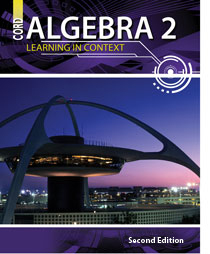# Algebra 2 - 2nd Edition

## Chapter 10: Trigonometric Functions and Identities

Some links are repeated for use with more than one lesson.

### The following links are applicable to the entire chapter.

http://www.explorelearning.com/index.cfm?CourseID=261&method=cResource.dspResourcesForCourse
This page contains interactive gizmos on various aspects of trigonometry. This website requires Shockwave Player to run some of its features.

http://www.mathdemos.org/mathdemos/family_of_functions/trig_gallery.html
This page contains a series of animations on trig functions and their inverses.

### 10.1 Right Triangle Trigonometry

http://www.themathpage.com/aTrig/trigonometry-of-right-triangles.htm

http://www.math.com/homeworkhelp/Trigonometry.html

### 10.2 Evaluating Trigonometric Functions

http://scherer.wikispaces.com/The+Unit+Circle
This page presents a nice graphic showing many special angles and the trigonometric values on the unit circle.
Because of the way this page redirects, you may find it necessary to copy and paste the url into your browser to avoid a page error.

http://www.mathsisfun.com/algebra/trig-interactive-unit-circle.html
This page has an interactive unit circle, instantly giving the values and graphs for the three trig functions.

### 10.3 Inverse Trigonometric Functions

http://demonstrations.wolfram.com/DomainAndRangeOfInverse
TrigonometricFunctions/

This page contains a graphing applet that will graph a function and its inverse.

http://www.regentsprep.org/Regents/math/algtrig/ATT8/indexATT8.htm
This series of pages discusses the inverse trig functions and their graphs.

### 10.4 Graphing Trigonometric Functions

http://www.purplemath.com/modules/grphtrig.htm
This series of pages discusses the graphing of trig functions, including the impact of real-world parameters of amplitude, frequency, and phase shift.

http://www.regentsprep.org/Regents/math/algtrig/ATT7/indexATT7.htm
This series of lessons provides an in-depth look at graphing the trig functions, beginning with terminology and including practice problems.

### 10.5 The Law of Sines and Law of Cosines

http://hyperphysics.phy-astr.gsu.edu/hbase/lsin.html
This page contains a calculator and applications of the Law of Sines.

http://www.wisc-online.com/objects/index_tj.asp?objID=TMH2702

http://www.college-cram.com/study/trigonometry/presentations/292

http://www.mathsisfun.com/algebra/trig-solving-triangles.html
This site includes practical examples and lessons on solving for unknown parts of triangles, including the Laws of Sines and Cosines.

### 10.6 Verifying Trigonometric Identities

http://www.teachervision.fen.com/tv/printables/botr/botr_141_12-12.pdf
This page contains a free printable worksheet on using graphs to verify the identities.

http://www.mathsisfun.com/algebra/trig-magic-hexagon.html

### 10.7 Angle Sum and Difference Identities

http://www.mathsisfun.com/algebra/trigonometric-identities.html
This page introduce the trig identities, but concludes with the half-angle, and angle sum and difference identities, including practice problems.

http://www.regentsprep.org/Regents/math/algtrig/ATT14/indexATT14.htm
This series of lessons and practice deals with the trig angle-sum and difference identities, as well as the identities covered in the next lesson.

http://www.shmoop.com/trig-functions/trig-sum-difference-identities.html
This page steps through proofs of sum and difference identities, as a good example of how to approach other identities.

### 10.8 Double-Angle and Half-Angle Identities

http://www.sosmath.com/trig/douangl/douangl.html